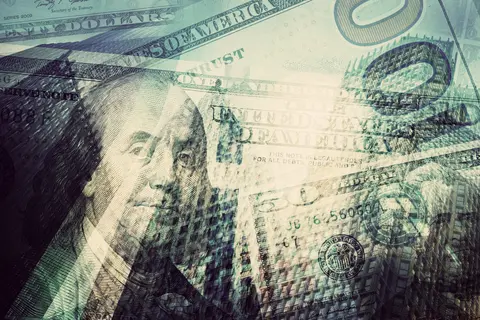# Reserve Ratio and Money Multiplier

The money multiplier refers to the way an initial deposit can lead to a larger final increase in the total money supply. Let’s say commercial banks gain deposits of \$1 million, which leads to a final money supply of \$10 million. In this example, the money multiplier is 10. This concept is a key element of the fractional banking system.

1. An initial increase in bank deposits (the monetary base) occurs.
2. The bank holds a fraction of the deposit and lends the rest of it.
3. The loan is re-deposited in banks, causing a further increase in lending and in the money supply.

## The Reserve Ratio

The percentage of deposits that banks hold in liquid reserves is the reserve ratio.

## The Formula for Money Multiplier

Theoretically, it’s possible to predict the size of the money multiplier if you know the reserve ratio.

• With a reserve ratio of 5%, a money multiplier of 1/0.05 or 20 is expected.
• The money multiplier of 20 is expected because if you have deposits of \$1 million and a reserve ratio of 5%, you can then lend out \$20 million.

## Example of Money Multiplier

• Let’s say that banks maintain a reserve ratio of 10%, or 0.1.
• If a person deposits \$100, the bank keeps \$10 as reserves and lends out the other \$90.
• Because \$90 has been lent out, other banks will see future deposits of \$90.
• As a result, the process of lending out deposits can start over.

Note: Although this example stops at stage 10, the process can theoretically continue for a long time, or until deposits are fractionally very small.

• If deposits can repeat infinitely, the final total deposits would be \$1000.
• The money multiplier is equal to 1/0.1, or 10.
• The final increase in the money supply is 10 multiplied by \$100, or \$1000.

## Using the Reserve Ratio to Influence Monetary Policy

If a Central Bank demands a higher reserve ratio, then it’ll act as a deflationary monetary policy. With a higher reserve ratio comes reduced bank lending and a reduction in the money supply.

## Money Multiplier in the Real World

When we talk about simple theories of the money multiplier, it’s assumed that if the bank lends \$90, all \$90 will return. In the real world, though, there are many reasons why the actual money multiplier is quite a bit smaller than the theoretical money multiplier.

1. Taxes: Taxes take a percentage of income.
2. Import Spending: Money leaves the economy when consumers buy imports.
3. Savings: A significant portion of the money is saved rather than spent and circulated.
4. Currency Drain Ratio: This refers to the percentage of banknotes that consumers keep in cash rather than depositing in the bank. There would be a larger money multiplier if consumers deposited all of their cash in banks. However, if people keep their funds in cash, the banks cannot lend more.
5. Bad Loans: If a bank lends \$90, but the company goes bankrupt, the \$90 is never deposited back into the system.
6. Safety Reserve Ratio: This refers to the percentage of deposits a bank wants to stay above the statutory reserve ratio. For instance, the required reserve ratio might be 5%, but banks may prefer to keep 5.2%.
7. It may not be possible to lend more money. Even if banks could lend out 95% of their deposits, that doesn’t mean they can in actuality, even if they wanted for. In the case of a recession, for example, people generally prefer to save rather than to borrow.
8. Banks may not wish to lend. There may be times that banks don’t want to lend. For instance, during a recession, banks may feel that individuals and firms are likely to default. Because of this, banks end up with higher reserve ratios.

Due to these factors, the money multiplier and reserve ratio are purely theoretical.

## Loan-First Multiplier

The model of the money multiplier suggests that banks first wait for a deposit and then lend out a fraction of it. But in the real world, banks might take it upon themselves to issue out a loan. Then they seek reserves from other financial institutions or private individuals.

One example is the credit bubble of 2000 to 2007. During this period, many banks lent mortgages by borrowing on short-term money markets. The money they were lending was not related to savings deposit accounts.

## Money Multiplier and Quantitative Easing

Between 2009 and 2012, Central Banks pursued quantitative easing, which involves growing the monetary base. They were able to attain greater cash reserves by buying bonds off banks. Theoretically, this increase in the money multiplier should also increase the overall money supply by a large amount.

But in practice, this didn’t take place. Since the banks weren’t keen to lend extra money, the money supply didn’t increase. Plus, banks were attempting to improve their reserves after the credit crunch and previous over-extension of loans.

Previous Post